Reach Us+447482877761
Synthesis, Morphology and Lead Ion Adsorption Properties of Metal Organic Frameworks of Copper and Cobalt | OMICS International
Chemical Sciences Journal
All submissions of the EM system will be redirected to Online Manuscript Submission System. Authors are requested to submit articles directly to Online Manuscript Submission System of respective journal.

# Synthesis, Morphology and Lead Ion Adsorption Properties of Metal Organic Frameworks of Copper and Cobalt

David Shooto N1*, Dixon Dikio E1, Wankasi D1 and Sikhwivhilu L2

1Applied Chemistry and Nano-Science Laboratory, Department of Chemistry, Vaal University of Technology, PO Box X021, Vanderbijlpark 1900, South Africa

2Advanced Materials Division, Mintek, Nanotechnology Innovation Centre, Private Bag X3015, Randburg 2125, South Africa

*Corresponding Author:
David Shooto N
Applied Chemistry and Nano-Science Laboratory
Department of Chemistry
Vaal University of Technology, PO Box X021
Vanderbijlpark 1900, South Africa
E-mail: [email protected]

Received Date: November 09, 2015; Accepted Date: November 12, 2015; Published Date: November 19, 2015

Citation: David Shooto N, Dixon Dikio E, Wankasi D, Sikhwivhilu L (2015) Synthesis, Morphology and Lead Ion Adsorption Properties of Metal Organic Frameworks of Copper and Cobalt. Chem Sci J 6:113. doi:10.4172/Chemical-Sciences.1000113

Copyright: © 2015 David Shooto N, et al. This is an open-access article distributed under the terms of the Creative Commons Attribution License, which permits unrestricted use, distribution, and reproduction in any medium, provided the original author and source are credited.

Visit for more related articles at Chemical Sciences Journal

#### Abstract

Metal organic frameworks based on Copper- and cobalt-1,2,4,5-tetrabenzenecarboxylates (Cu- and Co-MOFs) were synthesized, characterized and employed in metal adsorption studies. Characterization results were obtained from scanning electron microscopy, energy dispersive x-ray, thermogravimetric analysis and x-ray diffraction spectroscopy. The morphological features of Copper- and Cobalt-MOFs showed highly crystalline material as confirmed by the SEM micrographs. EDX spectra of both Metal organic frameworks also showed the presence of C, O and OH which may facilitate in creating charges and functionalities on the surface of the Metal organic frameworks for adsorption. Thermodynamic, equilibrium and isotherm batch adsorption experiments were carried out to determine concentration, time and temperature effects. The results obtained showed that Cu-MOFs were more effective adsorbent than Co- MOFs.

#### Keywords

MOFs; Heavy metals; Adsorption

#### Introduction

Heavy metal pollution is slowly increasing in the environment through improper disposal of industrial effluents from factories such as manufacturing of fertilizers, pesticides, dye, battery manufacturing, paint coatings and mining activities . Heavy metals such as lead, cadmium, cobalt, copper, chromium, zinc, etc, exhibit toxic effect and have relatively high density. High levels of lead in the environment could be threatening to human health, plants, soil quality and ecologic systems . Lead is a toxic metal, ranked as one of the most hazardous elements to human health, even in low concentrations . Therefore, efficient removal of lead ions from water is of great importance. When ingested it can damage nervous connections causing blood and brain disorders . Lead poisoning typically results from consumption of contaminated food or water, but it can also occur through accidental ingestion of contaminated soil, dust, or lead based paints .

Because these heavy metals are non-biodegradable they should be removed from water . Among various removal techniques, adsorption is the most extensively used because of its easy handling and low cost [7,8]. Hence, researchers have developed numerous adsorbents that can be used to remove lead ions in aqueous solution. Materials such as activated carbon [9,10], Fly ash [11,12], crab shell [13,14], coconut shell , zeolite [16,17], manganese oxide [18,19], rice husk [20,21], etc. have been used for the adsorption of lead ions in aqueous solution. However, many existing adsorbents have limitations such as, less-effectiveness due to low surface area . This has directed research to explore for innovative and novel adsorbents that could be more effective and have higher surface area.

Metal-organic frameworks (MOFs) are a new class of complex structured porous composites, composed of different varieties of central metal ions attached to rigid organic linkers of which most ligands used so far are aromatic or aliphatic polycarboxylates . This kind of architectural structure provides MOFs with organic functionality, high thermal and mechanical stability, large pore sizes, and high surface areas . Although MOFs constitute an important family of materials attracting attention for their many potential applications in areas of industrially importance areas . We have chosen rigid tetracarboxylate organic ligands, because these tetracarboxylic acids have been widely used for the construction of functional transition metal based MOFs . The coordination chemistry of these complexes has been studied but not their morphology and metal adsorption properties . The four carboxylic groups are expected to form metal coordination as well as provide large surface area for adsorption . The application of MOFs for the removal of heavy metals from aqueous solutions is still to be exploited.

In this work, we describe the synthesis of copper and cobalt MOF of benzene-1,2,4,5-tetracarboxylic acid, their morphology, and lead ion adsorption properties in terms of equilibrium and kinetic parameters of these MOFs.

#### Materials and Methods

Materials

N,N-Dimethylformamide [HCON(CH3)2, DMF, 99.8%; AnalaR], Cobalt(II)nitrate hexahydrate [Co(NO3)2.6H2O, 98%, Sigma- Aldrich], Copper(II)nitrate [Cu(NO3)2.3H2O, 99%, Sigma-Aldrich] 1,2,4,5-Tetrabenzenecarboxylic acid (H4TBCA), [C10H6O8, 96%, Sigma-Aldrich], Methanol [CH3OH, 99.9%; Sigma-Aldrich], Lead nitrate [Pb(NO3)2, 99%, Sigma-Aldrich].

All reagents were obtained from commercial sources and were used without further purification.

Synthesis procedure

Copper metal organic framework (Cu-MOF): Copper MOFs were synthesized by solvothermal method. 80 ml DMF was transferred into a round bottom flask, with (0.012 mol) Cu(NO3)2 and (0.012 mol) 1,2,4,5-tetrabenzenecarboxylic acid and mildly stirred. The solution is then refluxed for 2 h at 120°C while still stirring. Blue crystals were obtained and isolated by centrifuge and washed with methanol several times. The obtained crystals were then dried in oven at 40°C for 30 min and used for further experiments.

Cobalt metal organic framework (Co-MOF): The above procedure is repeated for the synthesis of Co-MOF. 80 ml DMF was transferred into a round bottom flask, with (0.012 mol) Co(NO3)2 and (0.012 mol) 1,2,4,5-tetrabenzenecarboxylic acid and mildly stirred. The solution refluxed for 2 h at 120 °C while still stirring. Pink crystals were obtained and isolated by centrifugation and washed with methanol several times. The obtained crystals were then dried in oven at 40 °C for 30 min and used for further experiments.

Lead solution preparation: Pb2+ stock solution (100 ppm) was prepared by dissolving 0.1 g Pb(NO3)2 in 1 liter of distilled water. Dilutions were made to 60, 40 and 20 ppm respectively.

Concentration effect: 0.1 g of copper-MOF was weighed, and placed into each of the 3 test tubes. 20 ml of metal ion solution with standard concentration of 20, 40 and 60 ppm from lead nitrate: Pb(NO3)2 were added to each test tube containing the weighed sample and equilibrated by rocking for 30 min and then centrifuged at 2500 rpm for 5 min and decanted. The supernatants were stored for lead ion (Pb2+) analysis using atomic adsorption spectrometer.

Time dependence studies: 0.1 g of the copper-MOF was weighed and transferred into each of the 3 test tubes. 20 ml of the metal ion solution with standard concentration of 60 ppm from Pb(NO3)2 was added to each test tube containing the weighed sample and equilibrated by agitation for each time intervals of 10, 20 and 30 min respectively. The powered sample suspension were centrifuged for 5 min at 2500 rpm and decanted. The supernatants were stored for lead ion (Pb2+) analysis using atomic adsorption spectrometer.

Temperature effect: 0.1 g of copper-MOF was weighed placed in 4 test tubes. 20 ml of the metal ion solution with standard concentration of 60 ppm from Pb(NO)3)2 was added to each test tube containing the weighed sample and equilibrated by rocking for 30 min at temperatures of 25, 40, 60 and 80°C respectively using water bath. The solution was immediately centrifuged at 2500 rpm for 5 min and then decanted. The supernatant were stored for lead ion (Pb2+) analysis using atomic adsorption spectrometer.

Same procedures as mentioned above was followed for cobalt- MOF to determine concentration, time and temperature effect.

Characterization: The chemical features of the as-prepared MOFs composite were examined by SEM, EDX, XRD, FTIR and TGA. The surface morphology and EDX measurements were recorded with a JOEL 7500F Emission scanning electron microscope. X-Ray Diffractometer (XRD), Shimadzu XRD 7000 was used to identify obtained sample. Thermogravimetric Analyzer (TGA), Perkin Elmer TGA 4000 was used, analyses were performed from 30 to 900°C at a heating rate of 10°C/min under a nitrogen atmosphere. Fourier transform infrared spectroscopy (FTIR), supplied by Perkin Elmer equipped with FT-IR/ FT-NIR spectrometer, spectrum 400. The measuring range extended from 4000 to 520 cm-1. After adsorption studies, Atomic Absorption Spectroscopy (AAS) Shimadzu ASC 7000 auto sampler was used to measure the remaining Pb2+ ions in the solution.

Data analysis

The uptake of heavy metal ions was calculated from the mass balance, which was stated as the amount of solute adsorbed onto the solid. It equal the amount of solute removed from the solution. Mathematically can be expressed in equation 1: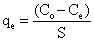(1)

Where

qe: Heavy metal ions concentration adsorbed on adsorbent at equilibrium (mg of metal ion/g of adsorbent).

Co: Initial concentration of metal ions in the solution (ppm).

Ce: Equilibrium concentration or final concentration of metal ions in the solution (ppm).

S: Dosage concentration and it is expressed by equation 2: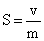(2)

Where ν is the initial volume of metal ions solution used in (L) and m is the mass of adsorbent.

The percent adsorption (%) was also calculated using equation 3: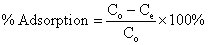(3)

Equilibrium studies

Langmuir plots were carried out using the linearized equation 4 below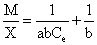(4)

Where X is the quantity of Pb2+ sorbed per mass M of the MOF in mg/g, a and b are the Langmuir constants obtained from the slope and intercepts of the plots.

Langmuir isotherm was showed in terms of an equation of separation factor or equilibrium parameter Sf.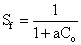(5)

Where, Co is the starting concentration of Pb2+ in aqueous solution, the value of the parameter Sf provides an indication of the type of sorption isotherm. If Sf>1.0, the sorption isotherm is unfavourable; Sf=1.0 (linear); 0<Sf<1.0 (favourable) and Sf=0 (irreversible).

The level of sorption of the Pb2+ in the MOF was measured from the Freundlich plots applying the linearized equation 6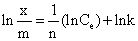(6)

Where, k and n are Freundlich constants and 1/n is approximately equal to the adsorption capacity.

The fraction of the MOF surface covered by the Pb2+ was calculated using equation 7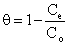(7)

With θ as degree of surface coverage

Thermodynamics studies

The capability of the adsorbent to reduce the amount of Pb2+ in solution was evaluated by the number of cycles of equilibrium sorption process needed according to the value of the partition coefficient (Kd) in equation 8.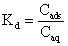(8)

Where, Caq is concentration of Pb2+ (mg/g) in solution; Cads is concentration of Pb2+ ppm) in MOF.

The isosteric heat of adsorption at constant surface coverage is calculated using the Clausius-Clapeyron equation: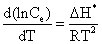(9)

Where, Ce is the equilibrium adsorbate concentration in the solution (ppm), Hx is the isosteric heat of adsorption (kJ mol-1), R is the ideal gas constant (8.314 J.mol-1K-1), and T is temperature (K). Integrating the above equation, assuming that the isosteric heat of adsorption is temperature independent, gives the following equation: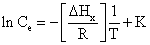(10)

Where, K is a constant.

The isosteric heat of adsorption is calculated from the slope of the plot of ln Ce versus 1/T.

The linear form of the modified Arrhenius equation was used to the experimental data to measure the sticking probability (S*) and activation energy (Ea) in equation 11.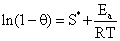(11)

The Gibbs free energy (ΔG°) of the adsorption was applied to evaluate the spontaneousness, was measured using the equation 12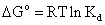(12)

Kd is derived from (Equation 8).

Further examination was done to measure the enthalpy (ΔH°) and entropy (ΔS°) of sorption by using equation 13.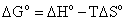(13)

The number of hopping (n) was calculated by relating it to the surface coverage (θ) in equation 14.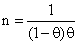(14)

Kinetic studies

To determine the kinetic compliance of the experimental data, the results were subjected to the following kinetic models:

Zero-order kinetic model,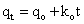(15)

where; qo and qt are the amounts of the adsorbed metal ion (mg/g) at the equilibrium time and at any instant of time “t”, respectively, and ko is the rate constant of the zero-order adsorption operation (l/min). Plotting of qt versus t gives a straight line for the zero-order kinetics.

First-order kinetic model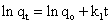(16)

Where,

k1 (min-1) is the rate constant,

qo (mg/g-1) is the amount of Pb2+ adsorbed on surface at equilibrium,

qt (mg/g-1) is the amount of Pb2+ adsorbed on surface at time t (min).

Plotting of Inqt versus t gives a straight line for the first-order kinetics.

Second-order kinetic model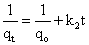(17)

Where,

k2 (min-1) is the rate constant,

qo (mg/g-1) is the amount of Pb2+ adsorbed on surface at equilibrium,

qt (mg/g-1) is the amount of Pb2+ adsorbed on surface at time t (min).

Plotting of 1/qt versus t gives a straight line for the second-order kinetics.

#### Results and Discussion

Scanning electron microscope images of copper and cobalt MOFs are presented in Figure 1a and 1b. The copper MOF image, Figure 1a, shows irregular shaped flakes of crystals while the cobalt MOF Figure 1b, show clusters of rectangular rod shaped material. The morphology of the as prepared MOFs composites was consistent with other SEM images published for these materials Copper-MOF  and Cobalt-MOF .

Figure 1: Scanning electron micrograph image of synthesized (a) Cu- MOF (b) Co-MOF obtained from copper (II) nitrate and cobalt (II) nitrate reacted with 1,2,4,5-Tetrabenzenecarboxylic acid respectively.

Elemental analysis of the as-prepared MOFs composites was investigated. The EDX spectrum in Figure 2a. Copper-MOF, showed the presence of multiple peaks corresponding to the following elements: C (72.6%), O (22.0%) and Cu (5.4%) whilst Figure 2b Cobalt-MOF: C (78.4%), O (18.4%) and Co (3.3%) as presented in Table 1. The presence of these elements creates negative charges on the surface of the as-prepared MOFs composites and result in electrostatic attraction between the MOFs and Pb2+ in the solution.

Figure 2: Energy Dispersive X-ray spectroscopy (EDX) of synthesized (a) Cu-MOF (b) Co-MOF obtained from copper (II) nitrate and cobalt (II) nitrate reacted with 1,2,4,5-Tetrabenzenecarboxylic acid respectively.

Cu-MOF Co-MOF
Element Atom, (%) Element Atom, (%)
Carbon (C) 72.6 Carbon (C) 78.4
Oxygen (O) 22.0 Oxygen (O) 18.4
Copper (Cu) 5.4 Cobalt (Co) 3.3
Total 100 Total 100

Table 1: Table of percentage weight of element and atom obtained from energy dispersive X-ray spectroscopy of Cu-MOF and Co-MOF.

To examine the thermal stability and determine the weight loss of the MOF composites taking place in an inert atmosphere, TGA and DTA analysis were carried out as shown in Figure 3a Copper- MOF and Figure 3b Cobalt-MOF. In Figure 3a TGA and DTA profiles showed multiple decomposition steps. First decomposition was observed between 32-110°C as confirmed by DTA plot, this is due to loss of guest molecules such as physically adsorbed moisture, water molecules, methanol that was introduced during washing and organic solvent material DMF trapped in the cages of Copper- MOF (TGA plot showed a percentage weight loss of 1.41%). Second decomposition was the most intense, it occurred between 120- 430°C as shown by DTA plot this weight loss corresponds to the disintegration of the copper-MOF, first it lost its side bonds, H bond between Copper-MOF molecules and O bond between C=O. Finally the breaking of main bonds of Copper-MOFs (TGA plot showed a percentage weight loss of 82.1%). Beyond 430°C DTA plot recorded no weight loss.

Figure 3: Thermalgravimetric analysis (TGA) and Derivative of thermogravimetric analysis (DTA) of (a) Copper Metal-Organic Framework and (b) Cobalt Metal-Organic Framework.

Also in Figure 3b, TGA and DTA plots showed multiple decomposition steps. First weight loss was observed on the DTA plot between 35-120°C, this is due to the loss of absorbed moisture and water molecules present, methanol and DMF (TGA plot showed a percentage weight loss of 25.83%) Second decomposition between 200-550°C as shown by DTA plot the weight loss corresponds to the gradual break down of the cobalt-MOF (TGA plot showed a percentage weight loss of 79.15%). Beyond this point DTA plot recorded no weight loss.

The X-ray diffraction patterns are shown in Figure 4a and 4b. Figure 4a shows the diffraction peaks of copper-MOFs, which are indexed to 9.9°, 12.1°, 21.7°, 26.5°, 34.2°, 36.5° and 44.6°. The obtained copper- MOF XRD pattern is in agreement with the previously reported results . The XRD pattern of cobalt MOF is shown in Figure 4b. The XRD peaks are indexed to 9.8°, 17.2°, 26.2°, 41.8° and 79.1°. The obtained XRD diffractogram is in agreement with the previously reported results for this material . A sharp peak below 10° (2theta) is observed in both MOFs this is an indicates that the obtained materials are highly crystalline .

Figure 4: X-ray diffraction (XRD) spectra’s of (a) Copper Metal-Organic Framework and (b) Cobalt Metal-Organic Framework.

In Figure 5 present the plot of percentage adsorption of Pb2+ by Copper-MOF and Cobalt-MOF at various temperatures. Copper-MOF curve shows, the removal percentage of Pb2+ as follows 66.55%, 79.15%, 82.12%, 83.79% at 25, 40, 60 and 80°C respectively. The adsorption of Pb2+ was observed to be enhanced by the increase in temperature. This improvement could be due to increased kinetic energy of adsorbate and formation of new binding sites on the surface of Copper-MOF. While Cobalt-MOF plot showed a maximum adsorption of 74.14% at 40 °C after which further increase in temperature resulted in no significant increase in adsorption, equilibrium point was reached. This implies that Cobalt-MOF is not temperature depended.

Figure 5: Effect of temperature (o C) on lead adsorption by Cu-MOF (-â��-) and Co-MOF (-•-).

Isosteric heat of adsorption ΔHx is one of the basic requirements for the characterization and optimization of an adsorption process and is a critical design variable in estimating the performance of an adsorptive separation process. Plots of In Ce against 1/T in Figure 6a and 6b gives a slope equal to ΔHx. Below 80 KJ/mol is physical adsorption and between 80-400 KJ/mol is chemical adsorption [31,32]. The values of ΔHx derived from equation 10 was 118.15 and 118.32 KJ/mol for Copper- and Cobalt-MOF respectively which indicates that chemical adsorption mechanism occurred.

Figure 6: Plot of In Ce vs. 1/T for adsorption of Pb2+ onto (a) Cu-MOF and (b) Co-MOF.

The activation energy (Ea) and the sticking probability S* were calculated from equation 11, the values shown in Table 2 for Copper- MOF, Ea and S* are (-17.94 KJ/mol) and (0.1889), for Cobalt-MOF (-12.09 KJ/mol) and (0.2850) respectively as shown in Figure 7a and 7b. Negative activation energy (-Ea) indications the absence of energy barrier to cause the adsorption it occur and so adsorption exothermic. The sticking probability S* indicates the measure of the potential of an adsorbate to remain on the adsorbent. It is often interpreted as S*>1 (no sorption), S*=1 (mixture of physic-sorption and chemisorption), S*=0 (indefinite sticking – chemisorption), 0<S*<1 (favourable sticking – physic- sorption).

Figure 7: Plot of In(1-θ) vs. 1/T for adsorption of Pb2+ onto (a) Cu-MOF and (b) Co-MOF.

T, K ΔG°, KJ/mol ΔH°, KJ/mol ΔS°, J/molK Ea, KJ/mol ΔHx, KJ/mol
Cu-MOF 298 -2.65 18.72 61.92 -17.94 118.15
313 -2.79
333 -2.97
353 -3.14
Co-MOF 298 -2.27 2.90 15.83 -12.09 118.32
313 -2.38
333 -2.53
353 -2.69

Table 2: Thermodynamic parameters of the adsorption of Pb2+ onto Cu- and Co- MOF.

The probability of Pb2+ finding vacant site on the surface of the Copper- and Cobalt-MOF during the sorption was correlated by the number of hopping (n) done by the Pb2+ is calculated from equation 14. The hopping number is 7 and 5 for Copper- and Cobalt-MOF respectively. The lower the hopping number, the faster the adsorption. The low value of n obtained in this study suggests that the adsorption of Pb2+ on both MOFs was very fast.

In Table 2 also presents the Gibbs free energy ΔG° for the sorption of Pb2+ by the MOFs which was calculated from equation 12. Gibbs free energy is the fundamental criterion of spontaneity. The values of ΔG° indicates that the sorption process was spontaneous. The values of the enthalpy change (ΔH°) and entropy change (ΔS°) were calculated from equation 13 to be 18.72 KJ/mol and 61.92 J/molK for Copper-MOF and 2.90 KJ/mol and 15.83 J/molK for Cobalt-MOF respectively as shown in Figure 8a and 8b. A plot of ΔG° against T gives a straight line graph with slope and intercept defining the ΔH° and ΔG°. A positive ΔH° suggests that sorption proceeded favourably at a higher temperature and the sorption mechanism was endothermic. A positive ΔS° suggests that the freedom of the adsorbed Pb2+ was not restricted in the MOFs, indicating that physic-sorption mechanism occurred.

Figure 8:Plot of ΔGo vs. T for adsorption of Pb2+ onto (a) Cu-MOF and (b) Co-MOF.

The time dependent study reveals the fast kinetics process of adsorption of Pb2+ and shows the amount of time needed for maximum adsorption to occur. The variation in percentage removal of Pb2+ with time has been presented in Figure 9. Copper-MOF plot showed a maximum of 80.91% removal of Pb2+ was observed in 30 minutes and Cobalt-MOF 72.18% in 20 minutes. The relatively short contact time required to attain equilibrium suggests that a rapid uptake of Pb2+ by Copper- and Cobalt-MOF occurred to fill some of the vacant sites.

Figure 9: Time depended studies (min) on lead adsorption by Cu-MOF (-â��-) and Co-MOF (-♦-).

The experimental data for Copper-MOF fitted only second-order kinetic model, Figure 12a (R2=0.9908). Zero-order kinetic model, Figure 10a and first-order kinetic model, Figure 11a gave (R2=0.9781) and (R2=0.9817) respectively. Cobalt-MOF also fitted second-order kinetic model, Figure 12b (R2=0.9643) only. Zero-order kinetic model, Figure 10b (R2=0.8929) and first-order kinetic model, Figure 11b (R2=0.871).

Figure 10: Plot of qt vs. time for adsorption of Pb2+ onto (a) Cu-MOF and (b) Co-MOF.

Figure 11: Plot of In qt vs. time for adsorption of Pb2+ onto (a) Cu-MOF and (b) Co-MOF.

The percentage sorption of Pb2+ by the MOFs at different concentrations of the Pb2+ is presented in Figure 13. Copper-MOF, the maximum adsorption of 76.50% took place at concentration of 60 ppm Pb2+. While the maximum adsorption for Cobalt-MOF is 70.01% at concentration of 60 ppm Pb2+. This is because at lower concentration more MOFs pore spaces and adsorbing sites were available for the Pb2+ but as the concentration of Pb2+ increased, the adsorption capacity of both MOFs steadily decreased due to reduced availability of free pore spaces and adsorbing sites, reduction in adsorption capacity is noted from 40 ppm (Pb2+) solution and 60 ppm (Pb2+) solution the system was steadily reaching equilibrium.

Figure 13:Effect of concentration (ppm) on lead adsorption by Cu-MOF (-â��-) and Co-MOF (-♦-).

In Figure 14a and 14b shows the Langmuir isotherm plots of adsorption of lead ions by Copper- and Cobalt-MOF respectively. The results showed correlation coefficient (R2=1) for Cobalt-MOF Figure 4b, a straight line and therefore, the experimental data best fitted this isotherm model. Langmuir isotherm plot for Copper-MOF Figure 14a gave the lower value of correlation coefficient (R2=0.9868), which suggested that this model was not the best descriptor of Pb2+ ion adsorption.

Figure 14: Plot of M/X vs. 1/Ce for adsorption of Pb2+ onto (a) Cu-MOF and (b) Co-MOF.

The experimental data was further fitted in to confirm its applicability to the Freudlich model, the results as shown in Figure 15a and 15b, fitted perfectly Copper-MOF Figure 15a with correlation coefficient (R2=1). Figure 15b, Cobalt-MOF also gave the correlation coefficient (R2=0.9907), which suggested that the experimental data best fitted this isotherm model.

Figure 15: Plot of M/X vs. 1/Ce for adsorption of Pb2+ onto (a) Cu-MOF and (b) Co-MOF.

#### Conclusion

In this research, Copper- and Cobalt-MOFs were successfully synthesized. SEM micrographs showed crystalline materials of uneven shapes as sizes. The equilibrium and kinetic batch adsorption studies of Copper and Cobalt MOFs showed a remarkable fast adsorption for lead removal. Both MOFs were effective adsorbent for the removal of Pb2+ ions in aqueous solution. The results from this research will add to the knowledge base on the synthesis, characterization and the use of metal organic frameworks for sorption studies.

#### Acknowledgements

This work was supported by a research grant from the Faculty of Applied and Computer Science Research and Publications Committee of Vaal University of Technology.

#### References

Select your language of interest to view the total content in your interested language

### Article Usage

• Total views: 10713
• [From(publication date):
December-2015 - Sep 19, 2019]
• Breakdown by view type
• HTML page views : 10078

## Post your commentCan't read the image? click here to refresh
###### Peer Reviewed Journals

Make the best use of Scientific Research and information from our 700 + peer reviewed, Open Access Journals

International Conferences 2019-20

Meet Inspiring Speakers and Experts at our 3000+ Global Annual Meetings

Top